# NCERT Solutions for Class 8 Maths Chapter 1 Rational Numbers Ex 1.2

NCERT Solutions for Class 8 Maths Chapter 1 Rational Numbers Ex 1.2

### NCERT Solutions for Class 8 Maths Chapter 1 Rational Numbers Ex 1.2

NCERT Solutions for Class 8 Maths Chapter 1 Rational Numbers Exercise 1.2

Ex 1.2 Class 8 Maths Question 1.
Represent these numbers on the number line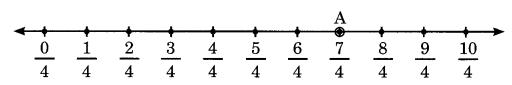Ex 1.2 Class 8 Maths Question 2.Ex 1.2 Class 8 Maths Question 3.
Write five rational numbers which are smaller than 2.
Solution:Ex 1.2 Class 8 Maths Question 4.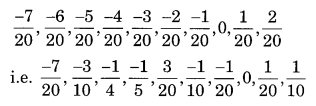Ex 1.2 Class 8 Maths Question 5.
Find five rational numbers between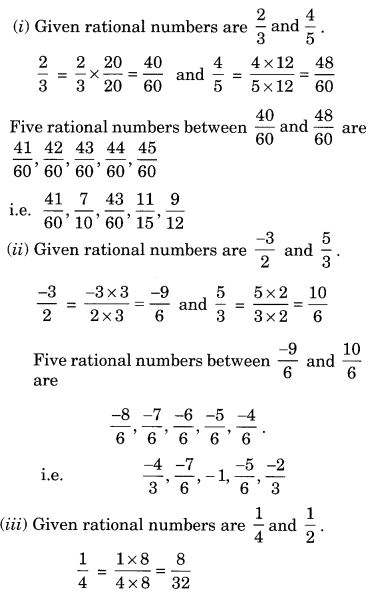Ex 1.2 Class 8 Maths Question 6.
Write five rational numbers greater than -2.
Solution: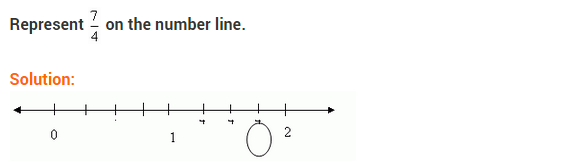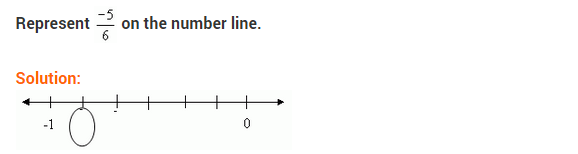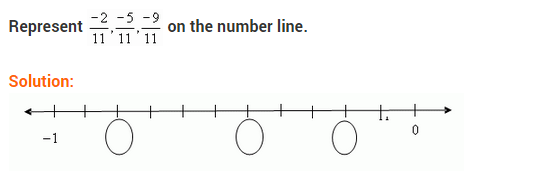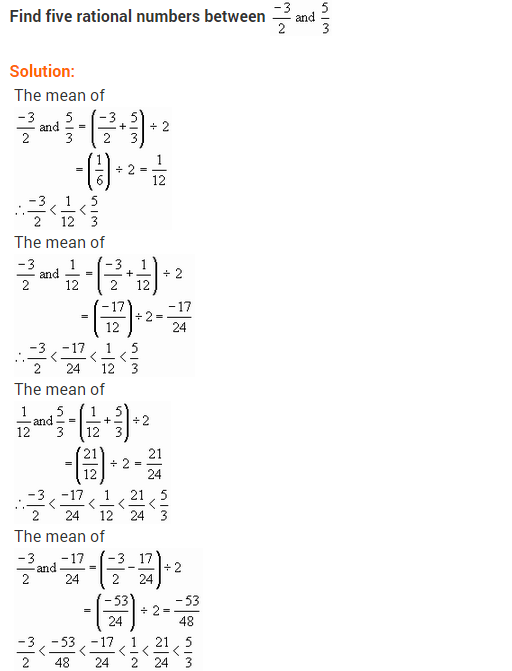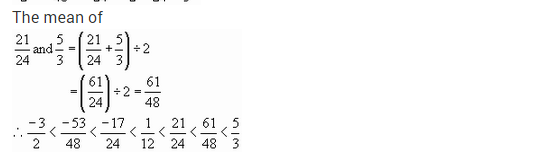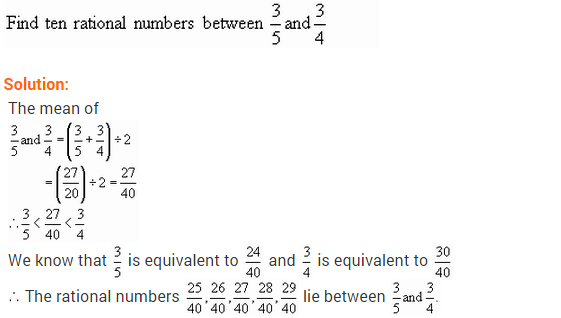## SabDekho

The Complete Educational Website